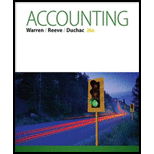# Using process costs for decision making The costs of materials consumed in producing good units in the Forming Department of Thomas Company were $76,000 and$77,350 for September and October, respectively. The number of equivalent units produced in September and October was 800 tons and 850 tons, respectively. Evaluate the change in the cost of materials between the two months.### Accounting (Text Only)

26th Edition
Carl Warren + 2 others
Publisher: Cengage Learning
ISBN: 9781285743615

#### Solutions

Chapter
Section### Accounting (Text Only)

26th Edition
Carl Warren + 2 others
Publisher: Cengage Learning
ISBN: 9781285743615
Chapter 20, Problem 20.8BPE
Textbook Problem
29 views

## Using process costs for decision makingThe costs of materials consumed in producing good units in the Forming Department of Thomas Company were $76,000 and$77,350 for September and October, respectively. The number of equivalent units produced in September and October was 800 tons and 850 tons, respectively. Evaluate the change in the cost of materials between the two months.

To determine

Process costs

It is a method of cost accounting, which is used where the production is continuous, and the product needs various processes to complete. This method is used to ascertain the cost of the product at each process or stage of production.

Equivalents units for production

The activity of a processing department in terms of fully completed units is known as equivalent units. It includes the completed units of direct materials and conversion cost of beginning work in process, units completed and transferred out, and ending work in process.

To Calculate: The equivalent cost per unit of direct material for the month of September.

### Explanation of Solution

Calculate equivalent cost per unit of energy for company T as shown below:

Equivalent cost per unit of direct materials for September) =Total direct materials cost during the period Equivalents units

To determine

To Calculate: The equivalent cost per unit of direct materials for the month of October.

To determine

To Evaluate: The changes in cost of energy between September and October.

### Still sussing out bartleby?

Check out a sample textbook solution.

See a sample solution

#### The Solution to Your Study Problems

Bartleby provides explanations to thousands of textbook problems written by our experts, many with advanced degrees!

Get Started

Find more solutions based on key concepts
What is the database model?

Accounting Information Systems

EXPECTED INTEREST RATE The real risk-free rale is 3%. Inflation is expected to be 2% this year and 4% during th...

Fundamentals of Financial Management, Concise Edition (with Thomson ONE - Business School Edition, 1 term (6 months) Printed Access Card) (MindTap Course List)

What are the three steps in decision making?

Pkg Acc Infor Systems MS VISIO CD